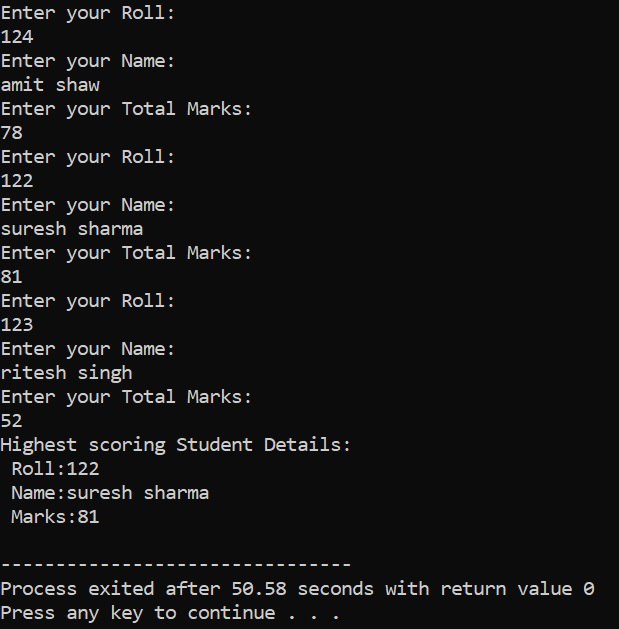# Passing array of objects as parameter in C++

• Difficulty Level : Basic
• Last Updated : 25 May, 2022

Array of Objects:It is an array whose elements are of the class type. It can be declared as an array of any datatype.

Syntax:

classname array_name [size];

Below is the C++ program to illustrate the array of objects by calculating the highest marks among 3 students:

## C++

 `// C++ program to illustrate the` `// passing the array of objects` `// to function parameter` `#include ` `using` `namespace` `std;`   `// Class Student` `class` `Student {` `    ``int` `roll;` `    ``char` `name;` `    ``int` `total;`   `public``:` `    ``// Function to input Roll Number` `    ``void` `getdata()` `    ``{` `        ``cout << ``"Enter your Roll: "` `             ``<< endl;` `        ``cin >> roll;` `        ``cout << ``"Enter your Name: "` `             ``<< endl;` `        ``cin.ignore();` `        ``cin.get(name, 50);` `        ``cout << ``"Enter your Total "` `             ``<< ``"Marks: "` `<< endl;` `        ``cin >> total;` `    ``}`   `    ``// Function to pass the array of` `    ``// objects` `    ``int` `pos(Student obj[], ``int` `size)` `    ``{` `        ``int` `pos = 0;` `        ``int` `max = obj.total;`   `        ``// Traverse the array of object` `        ``for` `(``int` `i = 0; i < size; i++) {` `            ``if` `(obj[i].total > max) {` `                ``max = obj[i].total;` `                ``pos = i;` `            ``}` `        ``}` `        ``return` `pos;` `    ``}`   `    ``// Function to display the students` `    ``// details` `    ``void` `putdata()` `    ``{` `        ``cout << ``"Roll: "` `<< roll` `             ``<< endl;` `        ``cout << ``"Name: "` `<< name` `             ``<< endl;` `        ``cout << ``"Total Marks: "` `             ``<< total << endl;` `    ``}` `};`   `// Function that have array of objects` `void` `arrayOfObjects()` `{` `    ``Student s, s1;` `    ``int` `pos;` `    ``for` `(``int` `i = 0; i < 3; i++) {` `        ``s[i].getdata();` `    ``}` `    ``pos = s1.pos(s, 3);` `    ``cout << ``"Highest scoring Student"` `         ``<< ``" Details:"` `<< endl;` `    ``s[pos].putdata();` `}`   `// Driver Code` `int` `main()` `{` `    ``// Function Call` `    ``arrayOfObjects();`   `    ``return` `0;` `}`

Output:Explanation:

• In the main() function objects of the Student class are created:
• Here, the first array of objects is s and the other one is s1(a simple object).
• In the for loop, 3 sets of user input have been taken, (i.e, it is where the user will be entering the name, roll, total marks, for 3 students sets).
• Then passing the s (array of an object which contains the sets of student details) and its size, through the s1 object in the pos(Student obj [], int size) member function.
• The pos(Student obj [], int size) function, is returning the position of the object of the highest total marks scoring student set, i.e, (0, 1or 2 index position of s object array), which is stored in pos = s1.pos(s, 3).
• Display part: For calling the display function, S[pos].putdata() is used.
• The putdata() function is displaying the object details of the student class.
• Here, pos is sent (which stores the index position of the highest student set object) in s, to display the highest total marks scored student details.

My Personal Notes arrow_drop_up
Recommended Articles
Page :